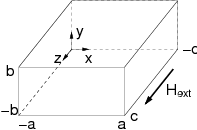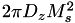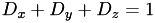# Calculator for magnetostatic energy and demagnetizing factor

DISCLAIMER/GPL TERMS AND CONDITIONS: see GNU General Public License

## Calculator for the magnetostatic energy and demagnetizing factor of a uniformly magnetized ferromagnetic prism

This tool (Javascript program) calculates the magnetostatic self energy and demagnetizing factor of a uniformly magnetized ferromagnetic rectangular prism analytically .Geometry of a regular prism with edge lengths 2a,2b,2c

This provides a quick and simple test if a micromagnetic code calculates the magnetostatic energy correctly. As input the 3 edge lengths of the prism and the magnetization saturation are required.

Dz is the demagnetizing factor when the body is magnetized uniformly along the z-axis.

 a [nm] b [nm] c [nm] Js [Tesla] demagnetizing factor Dz Energy density [kJ/m3] Energy [kB 300 K]

### Explanation

This calculator evaluates the following formula:The self energy per volume unit then becomes:Note that:JavaScript code developed, published under GPL, and contributed by Rok Dittrich.

 Amikam Aharoni, "Demagnetizing factors for rectangular ferromagnetic prisms"
J. Appl. Phys. 83 (1998) 3432 [ J. Appl. Phys. 1 ], [ J. Appl. Phys. 2 ]

magpar - Parallel Finite Element Micromagnetics Package